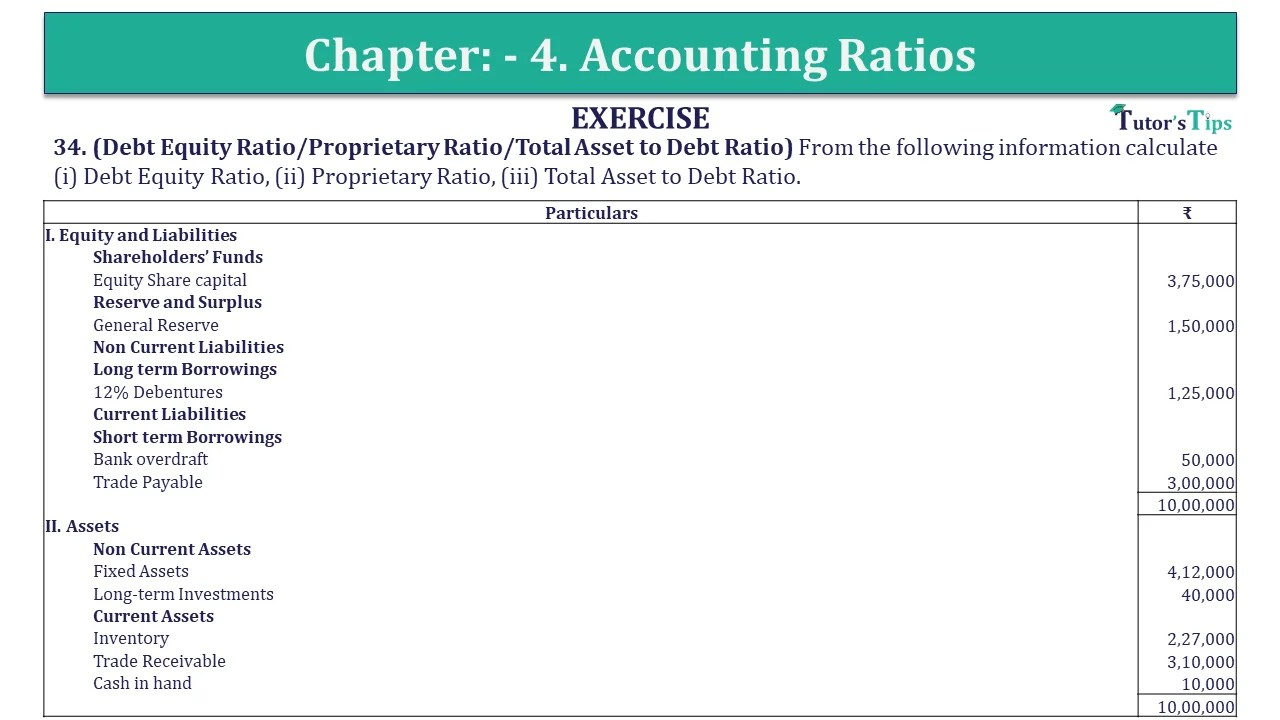# Question 34 Chapter 4 of +2-B – USHA Publication 12 ClassQ-34- CH-4 Book 2 - Usha Pub. +2 Book 2020 - Solution

Question 34 Chapter 4 of +2-B

34. (Debt Equity Ratio/Proprietary Ratio/Total Asset to Debt Ratio) From the following information calculate (i) Debt Equity Ratio, (ii) Proprietary Ratio, (iii) Total Asset to Debt Ratio.

 Particulars ₹ I. Equity and Liabilities Shareholders’ Funds Equity Share capital 3,75,000 Reserve and Surplus General Reserve 1,50,000 Non Current Liabilities Long term Borrowings 12% Debentures 1,25,000 Current Liabilities Short term Borrowings Bank overdraft 50,000 Trade Payable 3,00,000 10,00,000 II. Assets Non Current Assets Fixed Assets 4,12,000 Long-term Investments 40,000 Current Assets Inventory 2,27,000 Trade Receivable 3,10,000 Cash in hand 10,000 10,00,000

## The solution of Question 34 Chapter 4 of +2-B: –

 (i) Debt Equity Ratio = Total Long term Debt Shareholder’s Fund
 Total Long term Debt = 12% Debentures = ₹ 1,25,000 Shareholder’s Fund = Equity share Capital + General Reserve = ₹ 3,75,000 + ₹ 1,50,000 = ₹ 5,25,000
 Debt Equity Ratio = ₹ 1,25,000 ₹ 5,25,000 = 0.238: 1

 (ii) Proprietary Ratio = Proprietor’s Funds Total Assets
 Proprietor’s Funds = Equity share Capital + General Reserve = ₹ 3,75,000 + ₹ 1,50,000 = ₹ 5,25,000 Total Assets = Fixed Assets + Long Term Investments + Inventory + Trade Receivable +Cash at Bank = ₹ 4,12,500 + ₹ 40,000 + ₹ 2,27,500 + ₹ 3,10,000 + ₹ 10,000 = ₹ 10,00,000
 Debt Equity Ratio = ₹ 5,25,000 ₹ 10,00,000 = 0.525: 1

 (iii) Total Assets to Debt Ratio = Total Assets Long term Debt
 Total Assets = Fixed Assets + Long Term Investments + Inventory + Trade Receivable +Cash at Bank = ₹ 4,12,500 + ₹ 40,000 + ₹ 2,27,500 + ₹ 3,10,000 + ₹ 10,000 = ₹ 10,00,000 Long term Debt = 12% Debentures = ₹ 1,25,000
 (iii) Total Assets to Debt Ratio = ₹ 10,00,000 ₹ 1,25,000 = 8: 1

Also, Check out the solved question of previous Chapters: –